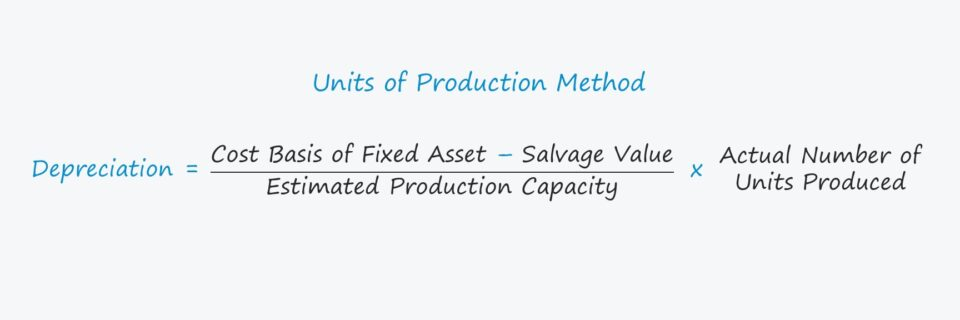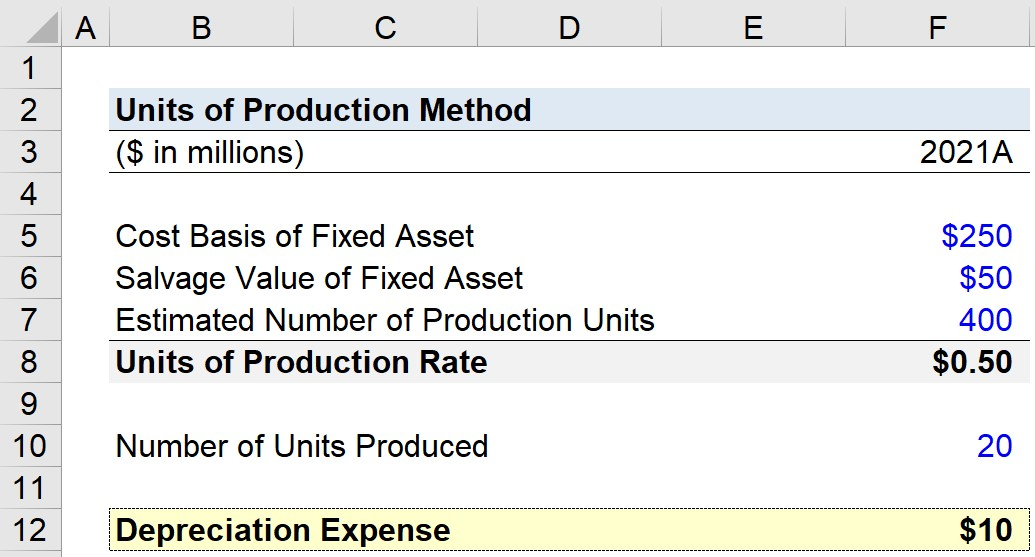Welcome to Wall Street Prep! Use code at checkout for 15% off.# Units of Production Method

Guide to Understanding the Units of Production Method## How to Calculate Units of Production Depreciation (Step-by-Step)

The units of production method attempts to recognize depreciation based on the actual “wear and tear” of the fixed asset on the balance sheet.

In comparison to the most common approach, the straight-line method, where the annual depreciation expense recorded equals the purchase price of the fixed asset minus the salvage value and divided by the useful life assumption, the units of production method is more complex yet more “accurate”, per se.

Instead of depreciating the asset on the basis of a useful life assumption, i.e. the number of years that the fixed asset is expected to provide positive economic benefits, the asset is depreciated based on its actual utilization and in terms of its remaining capacity.

• Periods with Greater Usage of Fixed Assets → Higher Depreciation Expense
• Periods with Less Usage of Fixed Asset Base → Lower Depreciation Expense

In effect, the depreciation expense recorded each year directly reflects how much of the fixed asset was used.

The steps to calculate the depreciation expense using the units of production method are the following:

• Step 1 → Estimate the useful life of the fixed asset in terms of the number of units produced, rather than in terms of years.
• Step 2 → Subtract the estimated salvage value, i.e. the residual value remaining at the end of the fixed asset’s useful life assumption, from the purchase price of the fixed asset
• Step 3 → Divide the estimated production capacity from the cost basis of the fixed asset net of the salvage value assumption, which results in the depreciation per unit of production.
• Step 4 → The depreciation expense recorded in the accounting period is the product of the number of units produced and the rate of depreciation per unit.

## Units of Production Method Formula

The formula to calculate the depreciation expense under the units of production method is as follows.

Depreciation Expense = [(Cost Basis of Fixed Asset – Salvage Value) ÷ Estimated Capacity of Total Units Produced] × Actual Number of Units Produced

## Limitations to the Units of Production Method

While more accurate in theory, the units of production method is more tedious and requires closely tracking the usage of the fixed asset.

However, the attempt to accurately depreciate an asset based on usage on a per unit basis also introduces more assumptions, resulting in more discretionary decisions (and more room for scrutiny from investors).

The question here becomes whether the marginal benefit of the added steps and granularity actually reflects financial performance more accurately (or if it is solely an attempt to be more accurate, without much of a material benefit).

In particular, the units of production method should not be used if usage of the fixed asset varies substantially each period because tracking the utilization of the asset will become a time-consuming task in itself.

Over the long run, the depreciation expense recorded is also unlikely to vary much from the amount recorded under the straight-line method, which is far more convenient and simpler to calculate.

Since financial statements are meant to be read and interpreted by investors to guide their decision-making, the added complexity is often not worth the effort or the time spent tracking the asset utilization, especially since the investors do not have access to all of the internal information.

Note: For tax purposes, the IRS does not prohibit the units of production method for recording depreciation, so the method’s primary use case is for internal bookkeeping.

## Units of Production Calculator — Excel Model Template

We’ll now move to a modeling exercise, which you can access by filling out the form below.Submitting...

## Units of Production Method Depreciation Calculation Example

Suppose a manufacturing company is tracking its depreciation expense under the units of production method.

At the end of fiscal year 2020, the company purchased a fixed asset, i.e. capital expenditure (Capex), for \$250 million.

According to management, the fixed asset has an estimated salvage value of \$50 million, and the total production capacity, i.e. the estimated number of total production units, is estimated at 400 million units.

• Cost Basis of Fixed Asset, BoP = \$250 million
• Salvage Value of Fixed Asset = \$50 million
• Estimated Number of Production Units = 400 Total Units

The units of production rate is equal to the depreciable fixed asset carrying value (i.e. the cost basis net of the salvage value assumption) divided by the estimated number of production numbers, which comes out to \$0.50.

• Units of Production Rate = (\$250 million – \$50 million) / (400 Units) = \$0.50

Thus, each unit produced depreciates the fixed asset by \$0.50.

If we assume that in 2021, a total of 20 million units were produced, we can arrive at the depreciation expense by multiplying our units of production rate by the actual number of units produced.

• Depreciation Expense = \$0.50 × 20 million = \$10 million

In closing, the estimated depreciation expense is calculated to be \$10 million for fiscal year ending 2021.Step-by-Step Online Course

### Everything You Need To Master Financial Modeling

Enroll in The Premium Package: Learn Financial Statement Modeling, DCF, M&A, LBO and Comps. The same training program used at top investment banks.